## July 1, 2019

### Structured Cospans

#### Posted by John BaezMy grad student Kenny Courser gave a talk at the 4th Symposium on Compositional Structures. He spoke about his work with Christina Vasilakopolou and me. We’ve come up with a theory that can handle a broad class of open systems, from electrical circuits to chemical reaction networks to Markov processes and Petri nets. The idea is to treat open systems as morphisms in a category of a particular kind: a ‘structured cospan category’.

Here is his talk:

In July 11th I’m going to talk about structured cospans at the big annual category theory conference, CT2019:

I borrowed more than just the title from Kenny’s talk… but since I’m an old guy, they’re giving me time to say more stuff. For full details, try Kenny’s thesis:

This thesis is not quite in its final form, so I won’t try to explain it all now. But it’s full of great stuff, so I hope you look at it! If you have any questions or corrections please let us know.

We’ve been working on this project for a couple of years, so there’s a lot to say… but right now let me just tell you what a ‘structured cospan’ is.

Suppose you have any functor $L \colon \mathsf{A} \to \mathsf{X}.$ Then a structured cospan is a diagram like this:

$\qquad$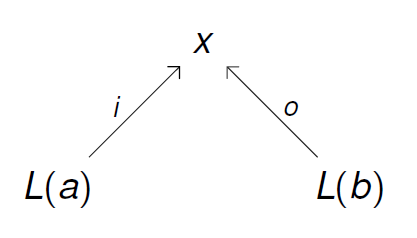For example if $L \colon \mathsf{A} \to \mathsf{X}$ is the functor from sets to graphs sending each set to the graph with that set of vertices and no edges, a structured cospan looks like this:

$\qquad$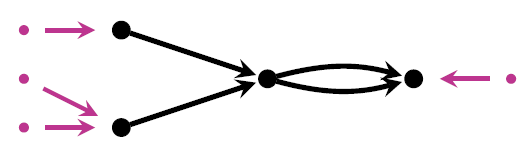It’s a graph with two sets getting mapped into its set of vertices. I call this an open graph. Or if $L \colon \mathsf{A} \to \mathsf{X}$ is the functor from sets to Petri nets sending each set to the Petri having that set of places and nothing else, a structured cospan looks like this:

$\qquad$It illustrates many ideas from the general theory of structured cospans: for example, what we do with them.

You may have heard of a similar idea: ‘decorated cospans’, invented by Brendan Fong. You may wonder what’s the difference!

Kenny’s talk explains the difference pretty well. Basically, decorated cospans that look isomorphic may not be technically isomorphic. For example, if we have an open graph like this:

$\qquad$and its set of edges is $\{a,b,c,d\},$ this is not isomorphic to the identical-looking open graph whose set of edges is $\{b,c,d,e\}.$ That’s right: the names of the edges matter!

This is an annoying glitch in the formalism. As Kenny’s talk explains, structured cospans don’t suffer from this problem.

My talk at CT2019 explains another way to fix this problem: using a new improved concept of decorated cospan! This new improved concept gives results that match those coming from structured cospan in many cases. Proving this uses some nice theorems proved by Kenny Courser, Christina Vasilakopoulou and also Daniel Cicala.

But I think structured cospans are simpler than decorated cospans. They get the job done more easily in most cases, though they don’t handle everything that decorated cospans do.

I’ll be saying more about structured cospans as time goes on. The basic theorem, in case you’re curious but don’t want to look at my talk, is this:

Theorem. Let $\mathsf{A}$ be a category with finite coproducts, $\mathsf{X}$ a category with finite colimits, and $L \colon \mathsf{A} \to \mathsf{X}$ a functor preserving finite coproducts. Then there is a symmetric monoidal category ${}_L \mathsf{Csp}(\mathsf{X})$ where:

• an object is an object of $\mathsf{A}$
• a morphism is an isomorphism class of structured cospans:

$\qquad$Here two structured cospans are isomorphic if there is a commutative diagram of this form:

$\qquad$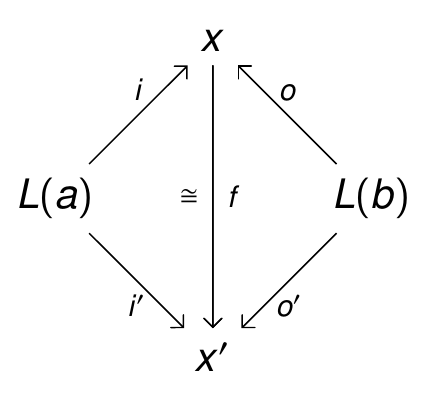If you don’t want to work with isomorphism classes of structured cospans, you can use a symmetric monoidal bicategory where the 1-morphisms are actual structured cospans. But following ideas of Mike Shulman, it’s easier to work with a symmetric monoidal double category. So:

Theorem. Let $\mathsf{A}$ be a category with finite coproducts, $\mathsf{X}$ a category with finite colimits, and $L \colon \mathsf{A} \to \mathsf{X}$ a functor preserving finite coproducts. Then there is a symmetric monoidal double category ${_L \mathbb{C}\!\mathbf{sp}(\mathsf{X})}$ where:

• an object is an object of $\mathsf{A}$

• a vertical 1-morphism is a morphism of $\mathsf{A}$

• a horizontal 1-cell is a structured cospan

$\qquad$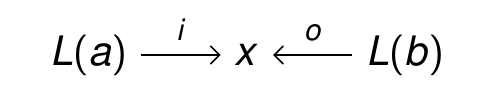• a 2-morphism is a commutative diagram

$\qquad$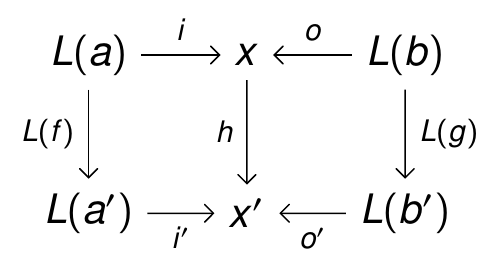Posted at July 1, 2019 7:39 PM UTC

TrackBack URL for this Entry:   https://golem.ph.utexas.edu/cgi-bin/MT-3.0/dxy-tb.fcgi/3123

### Re: Structured Cospans

Where’s the nlab pages for Decorated and Structured Cospans? I only see them mentioned on Comma Double Category (which I added myself).

Posted by: Max S. New on July 2, 2019 8:36 PM | Permalink | Reply to this

### Re: Structured Cospans

Max S. New wrote:

Where’s the nLab pages for Decorated and Structured Cospans?

Somewhere in the future, I guess. I suppose creating them would be an ingenious way for me to procrastinate on finishing the first paper on structured cospans. But I should really finish that, so maybe someone else can start the nLab pages.

Posted by: John Baez on July 4, 2019 8:46 PM | Permalink | Reply to this

### Re: Structured Cospans / Decorated cospans

This seems a nice definition. Thanks for the link to Kenny’s thesis, which looks really thorough.

I can’t shake off the following attempt at understanding decorated cospans. Maybe it is totally wrong, or maybe it connects to your perspective or something in Brendan or Kenny’s theses. I’m just trying to match it all to something more familiar.

Let $C$ have sums. The main ingredient for decorated cospans, a lax monoidal functor $F:C\to \mathbf{Set}$, is the same thing as a monoid $F$ in $\mathbf{Set}^C$. A monoid in any reasonable category induces a monad (free module), so in particular we have a monad $F\times (-)$ on $\mathbf{Set}^C$. It extends to a monad on $\mathrm{Span}(\mathbf{Set}^C)$ for some general reason, so we can consider the Kleisli (bi)-category for the monad. Now I think the category of $F$-decorated spans embeds faithfully in this Kleisli category, by the Yoneda embedding. That’s to say, to give a decorated cospan

(1)$c \to a \leftarrow d\quad 1\to F(a)$

is to give a span in $\mathbf{Set}^C$

(2)$y c \leftarrow y a \rightarrow (F\times y d)$

and the fancy composition of decorated spans is just Kleisli composition. (Here $y c=C(c,-)$ is the Yoneda embedding.) Is that right / reasonable?

Posted by: Sam Staton on July 4, 2019 10:10 AM | Permalink | Reply to this

### Re: Structured Cospans / Decorated cospans

Interesting. I’d have to do some calculating to check this. My first question is this. In the usual approach, it’s really obvious that the category of decorated cospans is a dagger-category. That is, there’s an obvious symmetry in the whole formalism, which maps $c \leftarrow a \rightarrow d$ to $d \leftarrow a \rightarrow c$. The Kleisli approach you describe obscures that. Is the symmetry still there, just hiding?

Posted by: John Baez on July 4, 2019 8:42 PM | Permalink | Reply to this

### Re: Structured Cospans / Decorated cospans

It’s a good question. It’s clear that the morphisms turn around, because a span $c \leftarrow a \rightarrow F\times d$ is the same thing as a morphism $a\to c\times F\times d$. But there’s a little bit of work to show that the composition is respected by this switch. Incidentally I think this is also a reasonable intuition towards showing that it’s also a compact closed category.

Posted by: Sam Staton on July 7, 2019 10:33 PM | Permalink | Reply to this

### Re: Structured Cospans

In Kenny’s most impressive thesis he mentions in the bibliography the 2005 TAC paper: R. Rosebrugh, N. Sabadini and R. F. C. Walters, “Generic commutative separable algebras and cospans of graphs”. Just want to mention a 16-page 2009 paper at arxiv that might be of interest, or at least deserve mention somewhere: L. de Francesco Albasini, N. Sabadini, R.F.C. Walters, “Cospans and spans of graphs: a categorical algebra for the sequential and parallel composition of discrete systems” https://arxiv.org/abs/0909.4136. The sadly now departed Bob Walters gives a little informal introduction to the paper in his blog: “On cospans and spans II”. As to the “Undefined control sequence” errors there, looking at the web page source shows the first two are just generic cospan and span triples, while the third is the natural 3x3 diagram in the paper’s Defn. 3.

Posted by: Keith Harbaugh on September 12, 2019 7:08 PM | Permalink | Reply to this

Post a New Comment